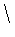Next: Type Predicates Up: The Prolog Language Previous: Control Annotation

# Arithmetic

Arithmetic in Prolog is, at most, pale in comparison with what is available in CLP systems, and resembles more what is available in common languages. It was designed not for constraint programming (which was not coupled with Prolog then), but rather for ease of implementation. The interface between arithmetics and the rest of the Prolog machinery is the evaluation of arithmetic terms. Certain terms (those constructed with designed functors, such as +/2, */2, etc., variables, and numeric constants), can be evaluated as arithmetic expression by some builtins, thus providing arithmetical operations. Table 4.1 shows some usually available functor names which are used to perform arithmetical operations, and Table 4.2 has some common builtins related to arithmetic. Note that functors are defined as operators, so that they can also be used infix / prefix.

Table 4.1: Some arithmetic-related terms
Functor Meaning
+/1 Positive prefix (usually unneeded)
-/2 Subtraction
-/1 Negative prefix
/ / 2 Division
// / 2 Integer division
mod/2 Module
log/1 Logarithm

Table 4.2: Some arithmetic-related builtins
Functor Rôle
is/2 Evaluation
=:= Arithmetic equality
== Arithmetic inequality
<, >, =<, >= Order relationship

Examples of correct arithmetical terms are 1 + 2, (56 // 4) mod (3 + 1), 45 / (X + 8) (if X is instantiated to a number at runtime; otherwise an error is raised). Syntactically incorrect arithmetical terms are, for example a + 3 (since a is not an arithmetical term), or X + (1 / (3 * f(o, H))).

The evaluation of arithmetical terms is performed via the predicate is/2: Z is T evaluates the arithmetical term T and the result is unified with the variable Z. If the unification fails, the predicate fails, and backtracking is forced. The following are examples of queries (which may be part of bodies of clauses):

```?- X is 3 + 5.
X = 8 ?

yes
?- X is 3 + 5, Y is X * X mod (X + 1).
X = 8, Y = 1 ?

yes
?- X is 3 + 5, Y is X * (X mod (X + 1)).
X = 8, Y = 64 ?

yes
?- X is 5 * (9 // 2), Y is (X * 2) // 3, X > Y.
X = 20, Y = 13 ?

yes
?- X is 5 * (9 // 2), Y is (X * 2) // 3, X =< Y.

no
?- X is 5 * (9 // g).
{ERROR: illegal arithmetic expression}

?- X is 32, X / 4 =:= (X // 3) -2.
X = 32 ?

yes
```

When an error is found, the system usually aborts execution, instead of failing.Next: Type Predicates Up: The Prolog Language Previous: Control Annotation
MCL
1998-12-03